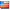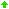Joined: 22 Sep 2008
United StatesLessons: 3
Licks: 5
Karma: 4# Diatonic Chords

`by Littlewing`

1 Jan 2009
Views: 13442

As another member requested, this lesson will be about the chords in a major scale.

Picture music as 12 equal boxes. Each on of these boxes is a note(or tone). The distance of a box to another box is a semitone. Two of these boxes is a whole tone. These boxes can be repeated in an octave with out end. Now we take seven of these boxes. Seven of these boxes represent the major scale. The formula for a major scale is WT WT ST WT WT ST. Now lets use C for example.
C D E F G A B would be the end result in using this formula. Any scale with 5 whole tones and 2 semitones is known as a Diatonic Scale.

A 3 note chord is known as a triad. Chords built off of a diatonic scale are called Diatonic Triads. Now we will find out the diatonic triads off a major scale.

Using C as an example, we have the notes C D E F G A B in a major scale. The formula for a major chord is 1(Your root tone), 3(Major third) and 5th(Perfect 5th). We look at C and we find the 3rd degree of the major scale which is E. Now we look at the 5th degree of the major scale and we get G. Now we have the chord C major. Of course C major isn't the only Triad that can be built from a C major scale right? Now we will apply this formula to D. The 2nd degree is a little bit different. The 2nd degree of a major scale will always be a minor chord. The formula for a minor chord is 1(Root) b3(Minor third) and 5th(Perfect 5th). Lets figure this out.

Now we count up a minor third from D. We get F. Now we count up a Perfect 5th from D and and we get A. This is your minor triad.

In a major scale, certain degrees of a major scale are assigned certain chords.

Root = Major
2nd = Minor
3rd = Minor
4th = Major
5th = Major
6th = Minor
7th = Minor flat 5 (1 b3 b5)

Hope this lesson has helped.

Until next time

Littlewing

Request:

#### Scale to Chord

by drm

C Major Scale for example, C D E F G A B C = 12345678. To get Cma7 chords there is 4 notes, C E G B = 1357 but is that the only chord that we could get from C Major Scale?